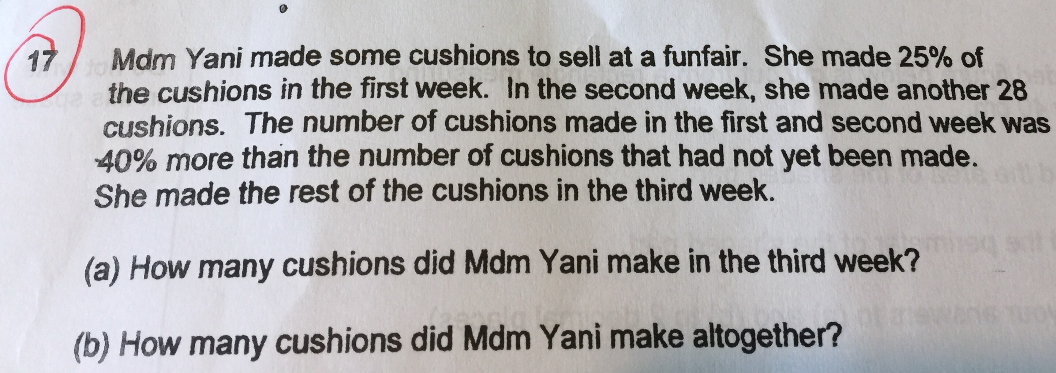# QuestionTargeted number of cushions 100% 1st week 25% 2nd week 28 3rd week Not yet made = 100% – 25% – 28                          = 75% – 28

Total cushions made in 1st and 2nd week = 25% + 28 (40% more than 3rd week)

100% + 40% = 140%

25% + 28 = 140/100 x (75% – 28)

25% + 28 = 105% – 39.2

105% – 25% = 39.2 + 28

80% = 67.2

1% = 67.2 ÷ 80

= 0.84

(a)

Cushions made in 3rd week = 75% – 28

= (75 x 0.84) – 28

= 63 – 28

= 35 (Ans)

(b)

100% = 0.84 x 100

= 84 (Ans)

0 Replies 0 Likes

(a)
first week ——- 25%
second week ——- 28
third week ——- 10u

100& – 25% = 75% ——- 28 + 10u
25% + 28 ——- 1.4 x 10u = 14u
75% ——- (14u – 28) x 3 = 42u – 84
42u – 84 ——- 28 + 10u
1u ——- (28 + 84)/(42 – 10) = 3.5
10u ——- 10 x 3.5 = 35

(b)
75% ——- 28 + 35 = 63
100% ——- (63 x 100/75) = 84

Ans : (a) 35 cushions; (b) 84 cushions.

0 Replies 1 Like